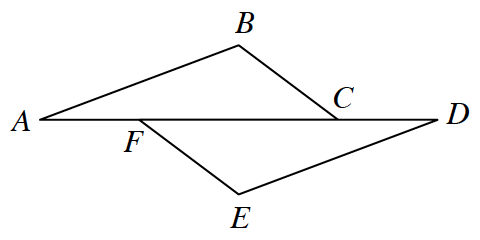### Home > CCG > Chapter 8 > Lesson 8.1.2 > Problem8-22

8-22.

Suzette started to set up a proof to show that if $\overline { B C } / / \overline { E F }$, $\overline { A B } / / \overline { D E }$ and $AF = DC$, then $\overline { B C } \cong \overline { E F }$. Examine her work below. Then complete her missing statements and reasons.Statements

Reasons

1. $\overline { B C } / / \overline { E F }$, $\overline { A B } / / \overline { D E }$ and $AF = DC$

1. $m\angle BCF = m\angle EFC$ and $m\angle EDF = m\angle CAB$

1. Reflexive Property

1. $AF + FC = FC + DC$

1. Additive Property of Equality (adding the same amount to both sides of an equation keeps the equation true)

1. $AC = DF$

1. $\Delta ABC \cong \Delta DEF$

1. $\cong\Delta\text{s}\rightarrow\;\cong\text{ parts}$

Statements

Reasons

1. $\overline{BC} // \overline{EF}, \overline{AB}// \overline{DE}$, and $AF = DC$

1. Given

1. $m\angle BCG = m\angle EFC$ and $m\angle EDF = m\angle CAB$

1. If two lines cut by a transversal are parallel, then alternate interior angles are equal.

1. $\boldsymbol{FC=FC}$

1. Reflexive Property

1. $AF + FC = FC + DC$

1. Additive Property of Equality (adding the same amount to both sides of an equation keeps the equation true)

1. $AC = DF$

1. $\Delta ABC \cong \Delta DEF$
1. $\textbf{ASA}\mathbf{\cong}$
1. $\boldsymbol{\overline{BC} \cong \overline{EF}}$
1. $\cong \Delta\text{s} \rightarrow \; \cong$ parts